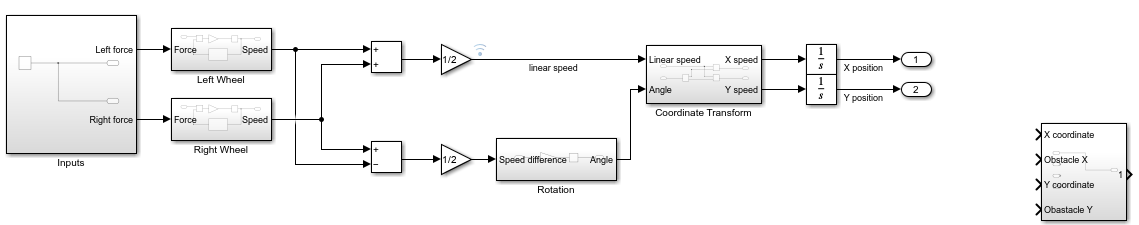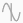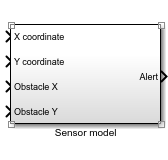## Design a System in Simulink

Model-Based Design paradigm is centered on models of physical components and systems as a basis for design, testing, and implementation activities. This tutorial adds a designed component to an existing system model.

### Open System Model

The model is a flat robot that can move or rotate with the help of two wheels, similar to a home vacuuming robot. Open the model by entering in the MATLAB® command line:

```open_system('system_model.slx') ```This tutorial analyzes this system and adds functionality to it.

### Identify Designed Components and Design Goals

Specification of the design objective is a critical first step to the design task. Even with a simple system, there could be multiple and even competing design goals. Consider these goals for the example model:

• Design a controller that varies the force input so that the wheels turn at a desired speed.

• Design inputs that make the device move in a predetermined path.

• Design a sensor and controller so that the device follows a line.

• Design a planning algorithm so that the device reaches a certain point using the shortest path possible while avoiding obstacles.

• Design a sensor and algorithm so that the device moves over a certain area while avoiding obstacles.

This tutorial designs an alert system. You determine the parameters for a sensor that measures the distance from an obstacle. A perfect sensor measures the distance from an obstacle accurately. An alert system samples those measurements at fixed intervals so that the output is always within 0.05 m of the measurement. The system generates an alert in time for the robot to come to a stop before hitting the obstacle.

### Analyze System Behavior Using Simulation

The design of the new component requires analyzing the linear motion of the robot to determine:

• How far the robot can travel at the top speed when power to the wheels is cut

• The top speed of the robot

Run the model with a force input that starts motion, waits until the robot reaches a steady velocity, and then sets the input force to zero:

1. In the model, double-click the Inputs subsystem.

2. Delete the existing step input and add a Pulse Generator block.

3. Set parameters for the Pulse Generator block:

• Amplitude: `1`

• Period: `20`

• Pulse Width: `15`

These parameters are designed to ensure that the top speed is reached. You can change parameters to see their effect.

4. Run the model for 20 sec.The first scope shows that the speed rapidly starts decreasing when the power is cut at time `3`. The speed then asymptotically approaches zero but does not quite reach it. This is a limitation of modeling; dynamics at low speeds without external force requires a more complex representation. For the objective here, however, it is possible to make approximations. Zoom in on the position signal.At time `3`, the position of the robot is at about 0.55 m. When the simulation ends, the position is less than 0.71 m. It is safe to say that the robot travels less than 0.16 m after the power is cut.

To find the top speed:

1. Zoom on the stable region of the velocity output in time, from 1 s to 3 s.

2. Leave zoom mode by clicking the zoom button again. Click the button.

3. Set the second cursor to the region where the velocity curve is flat.The Value column in the Cursor Measurements panel indicates that the top speed of the robot is 0.183 m/s. To calculate the time it takes for the robot to travel 0.05 m, divide 0.05 m by 0.183 m/s. You get 0.27 sec.

### Design Components and Verify Design

Sensor design consists of these components:

• Measurement of the distance between the robot and the obstacle — This example assumes that the measurement is perfect.

• The time interval at which the alert system measures the distance — To keep the measurement error below 0.05 m, this sampling interval must be less than 0.27 sec. Use 0.25 sec.

• The distance at which the sensor produces an alert — Analysis shows that slow down must start at 0.16 m from the obstacle. The actual alert distance must also take the error from discrete measurements, 0.05 m, into account.

Build the sensor:

1. Create a subsystem with the ports as shown.2. Construct the distance measurement subsystem. In the Sensor model block, use Subtract, Math Function with function `magnitude^2`, Sum, and Sqrt blocks as shown. Note the reordering of the input ports.3. Model sampling. Add a Zero-Order Hold block from the Discrete library to the subsystem and set the Sample time parameter to `0.25`.

4. Model the alert logic. Add a Compare to Constant block from the Logic and Bit Operations library and set the parameters:

• Operator: `<=`

• Constant Value: `0.21`

• Output data type: `boolean`

This logical block sets its output to `1` when its input is less than or equal to `0.21`.

5. Finish connecting the blocks.#### Verify Design

Test the design with an obstacle location of X = 0.65, Y = 0 using Constant blocks as inputs to the Sensor model subsystem. This test verifies design functionality in the X direction. You can create similar tests for different paths. This model only generates an alert. It does not control the robot.

1. Set the obstacle location. Add two Constant blocks from the Sources library set the constant values to `0.65` and `0`. Connect the position outputs of the robot to the inputs of the sensor.3. Run the model.Observe that the alert status becomes `1` once the position is within 0.21 m of the obstacle location and the design requirement for this component is satisfied.

For real-world systems with complex components and formal requirements, the Simulink® product family includes additional tools to refine and automate the design process. Requirements Toolbox™ provide tools to formally define requirements and link them to model components. Simulink Control Design™ can facilitate the design if you want to build a controller for this robot. Simulink Verification and Validation™ products establish a formal framework for testing components and systems.